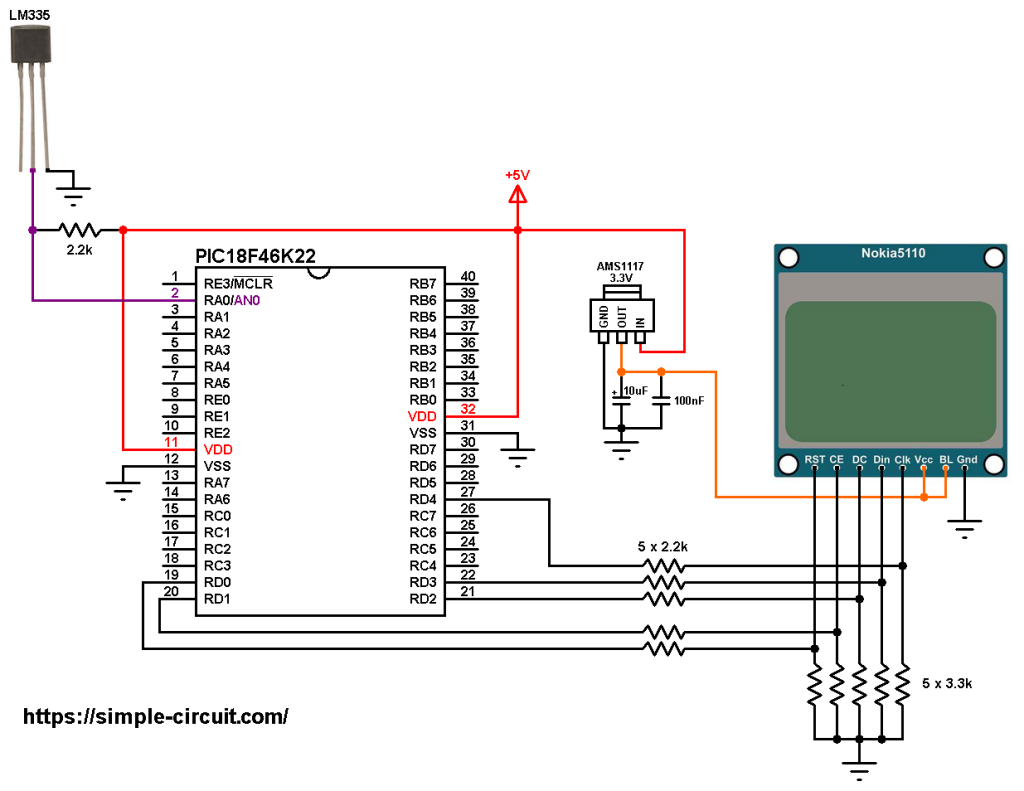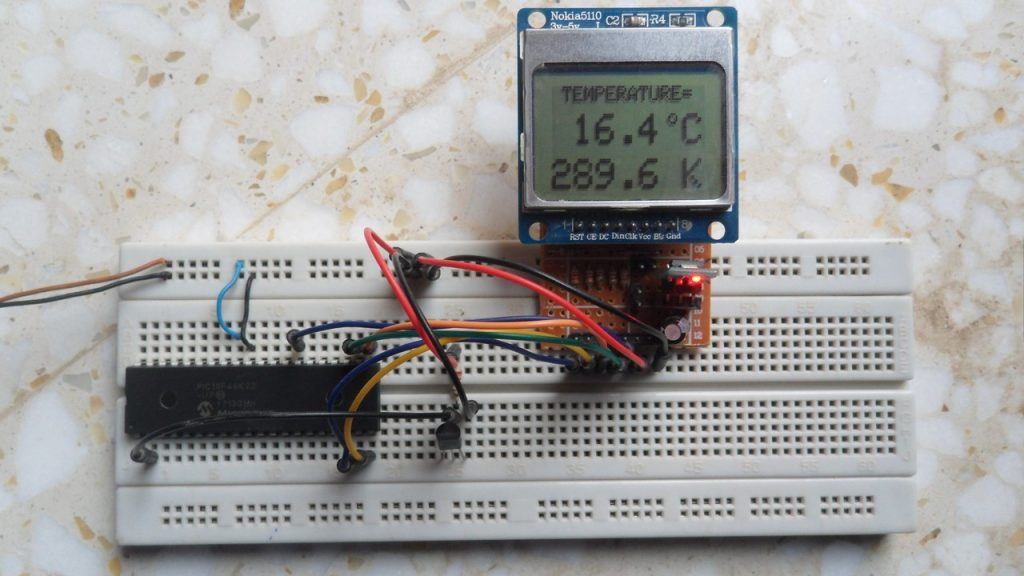# PIC18F46K22 MCU with Nokia 5110 LCD and LM335 sensor

This post shows how to build a temperature measurement station using PIC18F46K22 microcontroller and LM335 analog temperature sensor from Texas Instruments.
Nokia 5110 LCD (84×48 pixel) is used to display environment temperature in degree Celsius and Kelvin.
CCS C compiler is used in this project.

To see how to interface the PIC18F4550 with the Nokia 5110 using CCS C compiler, visit the post below: Interfacing PIC18F4550 MCU with NOKIA 5110 LCD

It’s is a 3-pin analog device which can measure temperature (converts temperature to analog voltage). This sensor requires an ADC module to convert the analog voltage into digital data. The PIC18F46K22 microcontroller has one ADC module with 10-bit resolution.

The LM335 sensor has the following features (from datasheet):

• Directly Calibrated to the Kelvin Temperature Scale
• 1°C Initial Accuracy Available
• Operates from 400 μA to 5 mA
• Less than 1-Ω Dynamic Impedance
• Easily Calibrated
• Wide Operating Temperature Range
• 200°C Overrange
• Low Cost

The LM335 has a breakdown voltage directly proportional to absolute temperature at 10 mV/°K. For example if the LM335 output voltage is equal to 3.03 (3030 mV) this means the temperature is: 303 Kelvin = 30 °Celsius.

The PIC18F46K22 is an 8-bit microcontroller that has a 10-bit ADC module. The good thing with this microcontroller is the fixed voltage references. With the fixed voltage reference we get a very good results.
Normally, negative and positive references of the ADC module are VSS and VDD, but VDD is not exactly equal to 5.00V and here we should use the fixed voltage reference as a positive reference of the ADC module.

The PIC18F46K22 has 3 fixed voltage references: 1.024V, 2.048V and 4.096V. For example if we set the fixed voltage reference to 4.096V and the ADC module is configured so that the negative and the positive references are VSS and FVR (Fixed Voltage Reference) respectively, in this case the equivalent 10-bit digital value of 4.096 is 1023 and 3.00V is 3.00 * 1023/4.096 = 749 , and so on.
In this project I used 4.096 because the LM335 output is between 2.23V (temperature = -50°C) and 3.98V (temperature = +125°C).

Hardware Required:

• PIC18F46K22 microcontroller   —->  datasheet
• Nokia 5110 LCD screen
• LM335 temperature sensor      —->   datasheet
• AMS1117 3V3 voltage regulator
• 10 uF capacitor
• 100 nF ceramic capacitor
• 5 x 3.3k ohm resistor
• 5 x 2.2k ohm resistor
• 2.2k ohm resistor
• 5V source
• Jumper wires

PIC18F46K22 MCU with Nokia 5110 LCD and LM335 sensor circuit:
The image below shows project circuit diagram.

The LM335 sensor has 3 pins (from left to right):
Pin 1 for calibration, not used in this example.
Pin 2: output.
Pin 3: GND (ground).

The output pin of the LM335 sensor is connected to pin RA0 which is analog channel 0 (AN0). I chose the 2.2k ohm because as written in the datasheet for optimum accuracy the current flows through the LM335 should be 1mA. For example if the temperature = 27°C, the output will be 3.00V and assume the supply voltage is exactly 5.00V that means the current flows through the sensor is ( 5 – 3)/2.2 = 0.90mA which is good enough. Also the value 2.2k is a standard value and well used.All the grounded terminals are connected together.

The Nokia 5110 which is shown in the circuit diagram has 8 pins (from left to right): RST (reset), CE (chip enable), DC (or D/C: data/command), Din (data in), Clk (clock), VCC (3.3V), BL (back light) and Gnd (ground).

The Nokia 5110 LCD works with 3.3V only (power supply and control lines). The LCD module is supplied with 3.3V which comes from the AMS1117 3V3 voltage regulator, this regulator steps down the 5V into 3.3V.

All PIC18F46K22 microcontroller output pins are 5V, connecting a 5V pin to the Nokia 5110 LCD may damage its controller circuit!
To connect the PIC18F46K22 to the LCD module, I used voltage divider for each line which means there are 5 voltage dividers. Each voltage divider consists of 2.2k and 3.3k resistors, this drops the 5V into 3V which is sufficient.

So, the Nokia 5110 LCD pins are connected to PIC18F46K22 MCU as follows (each one through voltage divider):
RST (reset) pin is connected to pin RD0 (#19)
CE (chip enable) pin is connected to pin RD1 (#20)
DC (data/command) pin is connected to pin RD2 (#21)
DIN (data in)  pin is connected to pin RD3 (#22)
CLK (clock) pin is connected to pin RD4 (#27)

VCC and BL are connected to AMS1117 3V3 regulator output pin and GND is connected to circuit ground (0V).

In this project the PIC18F46K22 microcontroller runs with its internal oscillator @ 16 MHz, MCLR pin is configured as an input pin.

PIC18F46K22 MCU with Nokia 5110 LCD and LM335 sensor C code:
The following C code is for CCS C compiler, it was tested with version 5.051.

To be able to compile the C code below with no error, a driver (library) for the Nokia 5110 LCD (PCD8544 controller) is required, its full name (with extension) is NOKIA5110.c, download link is the one below:

after the download of the library file, add it to project folder or CCS C compiler drivers folder.

Programming hints:
The connection of the LCD pins RST, CE, DC, DIN and CLK is defined in the code as shown below where software SPI is used:

Software SPI is initialized as shown below:

The internal fixed voltage reference (FVR) of the PIC18F46K22 microcontroller is set to 4.096V using the following line:

The ADC module is configured so that it uses its internal clock, voltage references (negative and positive) are set to VSS and FVR respectively where the FVR is the one previously set to 4.096V.
The LM335 output pin is connected to AN0 channel. All these configurations are done in the code as shown below:

PIC18F46K22 ADC module is used with 10-bit resolution which means the digital value of the input analog voltage varies between 0 (0V) and 1023 (4.096V). By multiplying the digital value by 4 we get the temperature in tenths Kelvin (4 = 1000*4.096/1024). The temperature in tenths degree Celsius = tenths Kelvin – 2732 (because: °C = K – 273.16).
To get the actual value of each quantity we’ve to divide it by 10. The line below shows an example for temperature in Kelvin:

We get the first 3 digits by dividing the tenths value by 10, and the tenths number (number after the decimal point) of the actual temperature value is equal to the reminder of that division (tenths value % 10).

The resolution of this thermometer is 0.4 °C.

CCS C Code:This site uses Akismet to reduce spam. Learn how your comment data is processed.